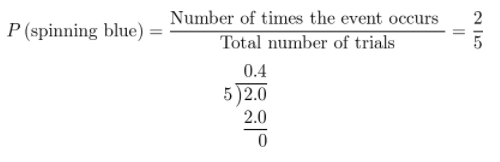Refer to our Texas Go Math Grade 7 Answer Key Pdf to score good marks in the exams. Test yourself by practicing the problems from Texas Go Math Grade 7 Module 5 Quiz Answer Key.

5.1 Probability

Question 1.
Josue tosses a coin and spins the spinner at the right. What are all the possible outcomes? __________________The possible outcomes are;
1. head and section 1 (red);
2. head and section 2 (green);
3. tails and section 1 (red);
4. tails and section 2 (green).

5.2 Experimental Probability of Simple Events

Question 2.
While bowling with friends, Brandy rolls a strike in 6 out of 10 frames. What is the experimental probability that Brandy will roll a strike in the first frame of the next game?The experimental probability that Brandy will roll a strike in the first frame of the next game is $$\frac{3}{5}$$

Question 3.
Ben is greeting customers at a music store. Of the first 20 people he sees enter the store, 13 are wearing jackets and 7 are not. What is the experimental probability that the next person to enter the store will be wearing a jacket?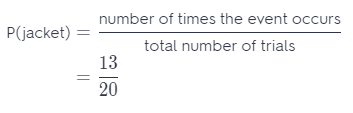The experimental probability that the next person to enter the store will be wearing a jacket is $$\frac{13}{20}$$.

5.3 Experimental Probability of Compound Events

Question 4.
Auden rolled two number cubes and recorded the results.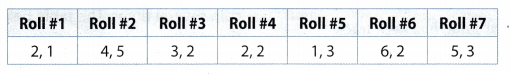What is the experimental probability that the sum of the next two numbers rolled is more than 5?
From the table we see that the number of times of event occurs is 3 (roll#2, roll#6 and roll#7).
P(the sum > 5) =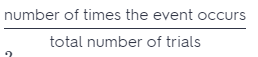= $$\frac{3}{7}$$
The experimental probability that the sum of the next two number rolled is more than 5 is $$\frac{3}{7}$$.

5.4 Making Predictions with Experimental Probability

Question 5.
A player on a school baseball team reaches first base $$\frac{3}{10}$$ of the time he is at bat. Out of 80 times at bat, about how many times would you predict he will reach first base?
Use a percent equation.
Find $$\frac{3}{10}$$ (30%) of 80.
Write 30% as a fraction. The percent equation will be
x = $$\frac{3}{100}$$ ∙ 80 Write fraction as decimal.
= 0.3 ∙ 80 Multiply.
= 24
About 24 times player will reach first base.

Essential Question

Question 6.
How is experimental probability used to make predictions?
Experimental probability is comparing the number of times the event occurs to the total number of trials Based on its name, experimentaL, this is the actual result of an experiment done. This is often used for small events which could be done through various trials. One example is when you want to determine the probability of getting a head or a tail when you flip a coin. In experimental probability, you could conduct a trial of flipping a coin 50 times.

The result will be the experimental probability.

Texas Go Math Grade 7 Module 5 Mixed Review Texas Test Prep Answer Key

Selected Response

Question 1.
A frozen yogurt shop offers scoops in cake cones, waffle cones, or cups. You can get vanilla, chocolate, strawberry, pistachio, or coffee flavored frozen yogurt. If you order a single scoop, how many outcomes are in the sample space?
(A) 3
(B) 5
(C) 8
(D) 15
(D) 15

Explanation:
There are 5 flavors of yogurt (vanilla, chocolate, strawberry, pistachio, coffee).
There are 3 ways to serve yogurt (cake cones, waffle cones, cups).
Hence, sample space is 3 × 5 = 15.

Question 2.
A bag contains 7 purple beads, 4 blue, beads, and 4 pink beads. What is the probability of not drawing a pink bead?
(A) $$\frac{4}{15}$$
(B) $$\frac{7}{15}$$
(C) $$\frac{8}{15}$$
(D) $$\frac{11}{15}$$
(D) $$\frac{11}{15}$$

Explanation:
P(pink bead) =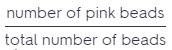P(pink bead) = $$\frac{4}{15}$$
The sum of the probabilities of an event and its complement equals 1
P(event) + P(complement) = 1
P(pink bead) + P(not pink bead) = 1 Substitute $$\frac{4}{15}$$ for P(pink bead)
$$\frac{4}{15}$$ + P(not pink bead) = 1 Subtract $$\frac{4}{15}$$ from both sides
P(not pink bead) = 1 – $$\frac{4}{15}$$
P(not pink bead) = $$\frac{11}{15}$$

Question 3.
During the month of June, Ava kept track of the number of days she saw birds in her garden. She saw birds on 18 days of the month. What is the experimental probability that she will see birds in her garden on July 1?
(A) $$\frac{1}{18}$$
(B) $$\frac{2}{5}$$
(C) $$\frac{1}{2}$$
(D) $$\frac{3}{5}$$
(D) $$\frac{3}{5}$$

Explanation:
P (Ava saw birds) == $$\frac{18}{30}$$ = $$\frac{3}{5}$$
The probability that she will see birds on July 1 is $$\frac{3}{5}$$

Question 4.
A rectangle has a width of 4 inches and a length of 6 inches. A similar rectangle has a width of 12 inches. What is the length of the similar rectangle?
(A) 8 inches
(B) 12 inches
(C) 14 inches
(D) 18 inches
(D) 18 inches

Explanation:
The rectangles are similar, hence, they have corresponding sides.
Write the proportion of the corresponding sides.The Length of rectangle is 18 inches.

Question 5.
The experimental probability of hearing thunder on any given day in Ohio is 30%. Out of 600 days, on about how many days can Ohioans expect to hear thunder?
(A) 90 days
(B) 180 days
(C) 210 days
(D) 420 days
(B) 180 days

Explanation:
Find 30% of 600.
Write 30% as a fraction. The percent equation will. be
x = $$\frac{30}{100}$$ ∙ 600 Write fraction as decimal
= 0.3 ∙ 600 Multiply.
= 180
Ohioans can expect to hear thunder about 180 days out of 600 days

Question 6.
Isidro tossed two coins several times and then recorded the results in the table below.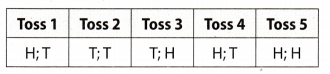What is the experimental probability that both coins will land on the same side on Isidro’s next toss?
(A) $$\frac{1}{5}$$
(B) $$\frac{2}{5}$$
(C) $$\frac{3}{5}$$
(D) $$\frac{4}{5}$$
(B) $$\frac{2}{5}$$

Explanation:
Total number of trials is 5: HT, TT, TH, HT, HH
N umber of time he tossed a same side on both coins is 2: TT, HH
Therefore, experimental probability that both of coins Land on same side in next toss is:
P (on both coins are same side) == $$\frac{2}{5}$$
Experimental probability that both of coins land on same side in next toss is $$\frac{2}{5}$$

Gridded Response

Question 7.
Magdalena had a spinner that was evenly divided into sections of red, blue, and green. She spun the spinner and tossed a coin several times. The table below shows the results.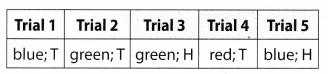Given the results, what is the experimental probability of spinning blue? Write an answer as a decimal.